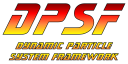#DPSFby Daniel Schroeder<PackageReference Include="DPSF" Version="2.5.0" />

# PivotPoint3D

public class PivotPoint3D
Class to hold and update an object's 3D Pivot Point (point to rotate around), Pivot Velocity, and Pivot Acceleration. This class requires a Position3D object, and optionally a Orientation3D object, that should be affected by rotations around the Pivot Point.
public Vector3 PivotPoint

The 3D Pivot Point that the object should rotate around.

NOTE: This only has effect when Rotational Pivot Velocity / Acceleration are used.

Get / Set the object's Rotational Acceleration around the Pivot Point (Position change).

NOTE: Rotations are specified by giving a 3D Vector, where the direction is the axis to rotate around, and the vector length is the amount (angle in radians) to rotate. It can also be thought of as Vector(PitchAcceleration, YawAcceleration, RollAcceleration).

public Vector3 PivotRotationalVelocity

The object's Rotational Velocity around the Pivot Point (Position change).

NOTE: Rotations are specified by giving a 3D Vector, where the direction is the axis to rotate around, and the vector length is the amount (angle in radians) to rotate. It can also be thought of as Vector(PitchVelocity, YawVelocity, RollVelocity).

public Orientation3D OrientationData { get; set; }

Get / Set the Orientation3D object that the Pivot Point should affect

public Position3D PositionData { get; set; }

Get / Set the Position3D object that the Pivot Point should affect

public bool RotateOrientationToo { get; set; }

Specify if the Update() function should Rotate the object's Orientation too when it rotates the object around the Pivot Point

public PivotPoint3D(PivotPoint3D cPivotPointToCopy)

Copy Constructor

public PivotPoint3D(Position3D cPosition)

Explicit Constructor. Set the Position3D object that should be affected by rotations around this Pivot Point.

public PivotPoint3D(Position3D cPosition, Orientation3D cOrientation)

Explicit Constructor. Set the Position3D and Orientation3D objects that should be affected by rotational around this Pivot Point.

public static Vector3 RotatePosition(Matrix sRotationMatrix, Vector3 sPivotPoint, Vector3 sPosition)

Returns the new Position after Rotating the given Position around the specified Pivot Point

public static void RotatePositionAndOrientation(Matrix sRotationMatrix, Vector3 sPivotPoint, ref Vector3 srPosition, ref Quaternion srOrientation)

Rotates the given Position and Orientation around the Pivot Point, changing the Position and Orientation

public void CopyFrom(PivotPoint3D cPivotPointToCopy)

Copy the given PivotPoint3D object's data into this object's data

public void RotatePosition(Matrix sRotationMatrix)

Rotates the object around the Pivot Point, changing its Position, without changing its Orientation

public void RotatePositionAndOrientation(Matrix sRotationMatrix)

Rotates the object about its center, changing its Orientation, as well as around the Pivot Point, changing its Position

public void Update(float fElapsedTimeInSeconds)

Update the Position and Orientation according to the Pivot Rotational Velocity / Acceleration